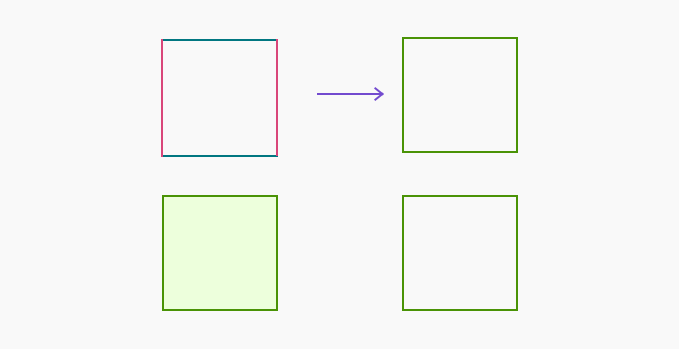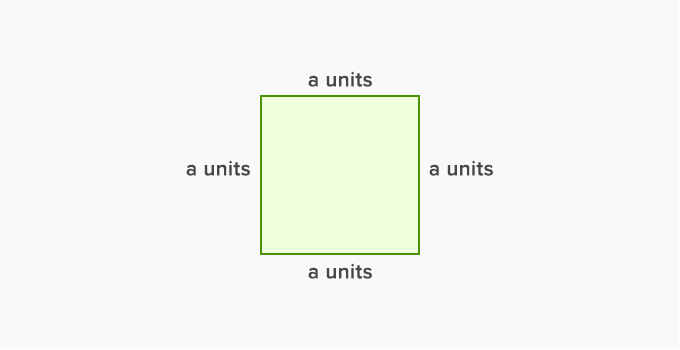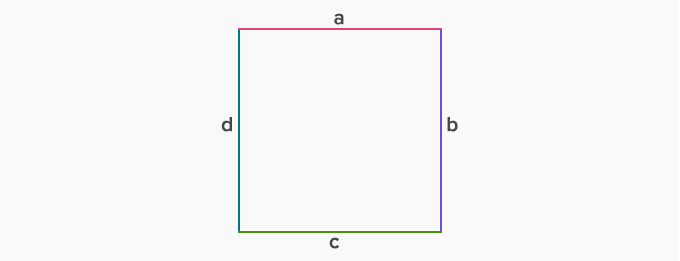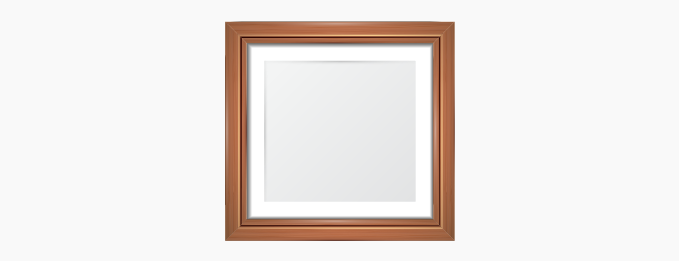Perimeter Of A Square - Definition with Examples

The Complete K-5 Math Learning Program Built for Your Child

• 30 Million Kids

Loved by kids and parent worldwide

• 50,000 Schools

Trusted by teachers across schools

• Comprehensive Curriculum

Aligned to Common Core

Perimeter of a Square

A square is a type of rectangle in which the adjacent sides are equal. In other words, all the sides of a square are equal.

Following are the properties of a square:

(i) All the angles in a square are the same and equal 90º.

(ii) All the sides of a square are equal.Perimeter of a square

The perimeter of a square is the total length of all the sides of the square. Hence we can find the perimeter of a square by adding all its four sides.The perimeter of the given square is a + a + a + a. Since all sides of a square are equal, we only need one side to find its perimeter.

The perimeter of the given square is:

a + a + a + a = 4  a units.

Hence, the formula of the perimeter of a square = 4 × (length of any one side).

Tricky math problems with the squares:

Type I: Finding side length when only the perimeter is given.

Example 1. If the perimeter of the given square is 12 cm. What will be the length of its side?Solution: Given, the perimeter of the square is 12 cm.

Let the side length be ‘a’ cm.

We know that, the perimeter of a square = 4 × (side length)

12=4×(a)

a = 3 cm

Type II: Finding sides using square properties.

Example 2.If a = 4 cm in the given square. Find b, c. and d.Solution: Given that side a = 4 cm.

To find side b, c, and d  we use the property of the square which states that all the sides of a square are equal.Therefore, a = b = c = d = 4 cm

Type III: Finding perimeter when one of the sides is given.

Example 3. One of the sides of a square is 5 cm. What will be its perimeter?Solution: Given, one side of the square is 5 cm.

We know that, the perimeter of a square = 4 × (side length)

= 4×(5)

= 20 cm

Example 4. The length of a side of the square wooden frame is 5 cm. Find the total length of the wood used in the frame.Solution: Given, the length of a side of this wooden frame is 5 cm.

As we know, the perimeter of a square = 4× (side length)

= 4  (5)

= 20 cm

Hence, the total length of the wood used is 20 cm.

 Fun Facts Squares are one of the three most basic shapes on the earth. The other two are circles and triangles.

Won Numerous Awards & Honors